# And Then There Was One

Time Limit: 5000MS

Memory Limit: 65536K

## Description

Let’s play a stone removing game.Initially, n stones are arranged on a circle and numbered 1, …, n clockwise (Figure 1). You are also given two numbers k and m. From this state, remove stones one by one following the rules explained below, until only one remains. In step 1, remove stone m. In step 2, locate the k-th next stone clockwise from m and remove it. In subsequent steps, start from the slot of the stone removed in the last step, make k hops clockwise on the remaining stones and remove the one you reach. In other words, skip (k − 1) remaining stones clockwise and remove the next one. Repeat this until only one stone is left and answer its number. For example, the answer for the case n = 8, k = 5, m = 3 is 1, as shown in Figure 1.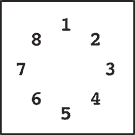Initial state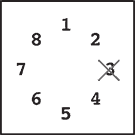Step 1Step 2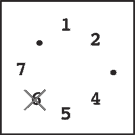Step 3Step 4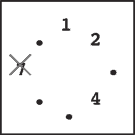Step 5Step 6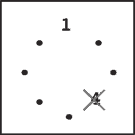Step 7Final state
Figure 1: An example game

## Input

The input consists of multiple datasets each of which is formatted as follows.n k mThe last dataset is followed by a line containing three zeros. Numbers in a line are separated by a single space. A dataset satisfies the following conditions.2 ≤ n ≤ 10000, 1 ≤ k ≤ 10000, 1 ≤ mnThe number of datasets is less than 100.

## Output

For each dataset, output a line containing the stone number left in the final state. No extra characters such as spaces should appear in the output.

## Sample Input

8 5 3
100 9999 98
10000 10000 10000
0 0 0

## Sample Output

1
93
2019

Japan 2007